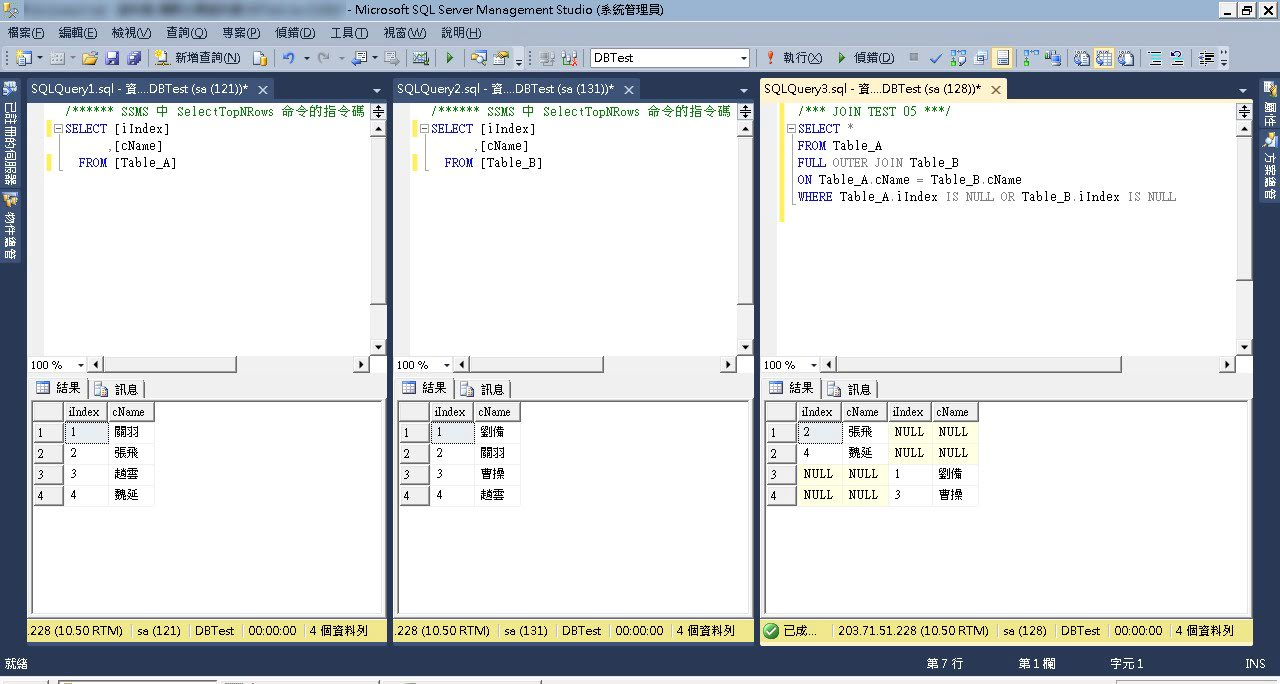﻿

# SQL筆記：SQL資料表之JOIN指令圖解介紹

``````SELECT [iIndex], [cName] FROM [Table_A]
---------------------------------------
iIndex  cName
1       關羽*
2       張飛
3       趙雲*
4       魏延

SELECT [iIndex], [cName] FROM [Table_B]
---------------------------------------
iIndex  cName
1       劉備
2       關羽*
3       曹操
4       趙雲*
``````

## 完整聯集：FULL OUTER JOIN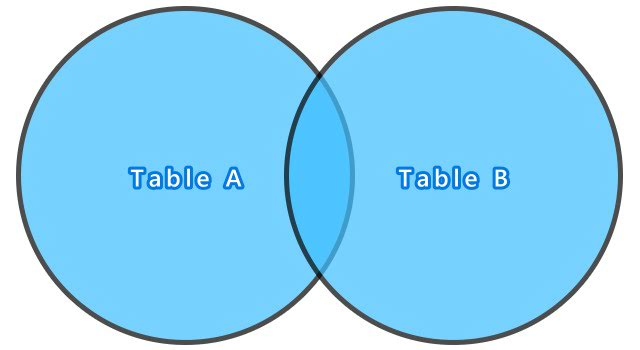``````使用指令與結果：
/*** JOIN TEST 01 ***/
SELECT *
FROM Table_A
FULL OUTER JOIN Table_B
ON Table_A.cName = Table_B.cName
``````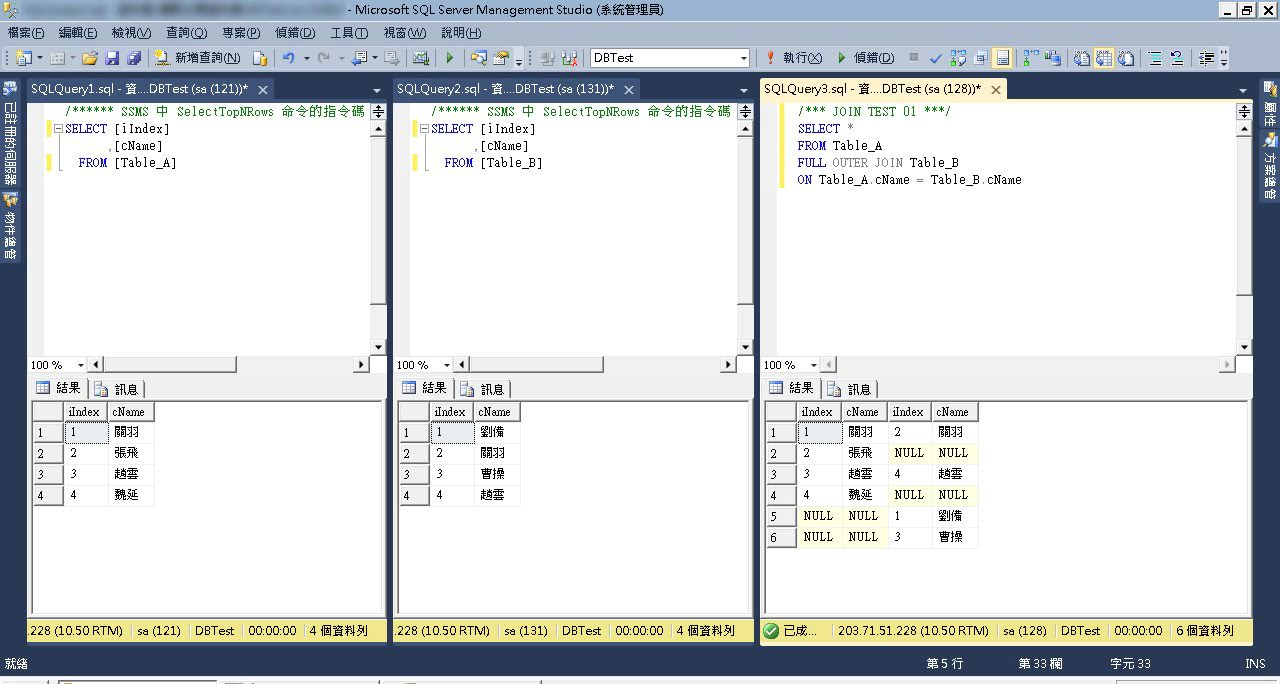## 完整交集：INNER JOIN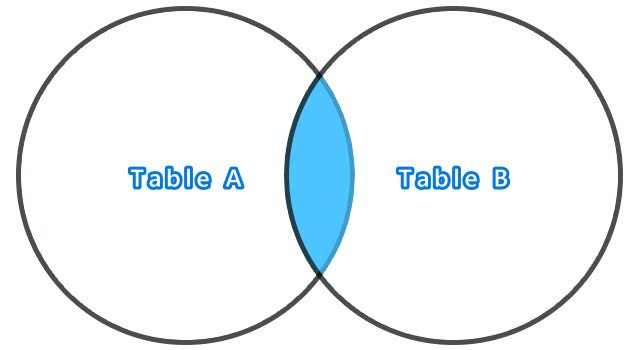``````使用指令與結果：
/*** JOIN TEST 02 ***/
SELECT *
FROM Table_A
INNER JOIN Table_B
ON Table_A.cName = Table_B.cName
``````## 部份聯集與完整交集：LEFT OUTER JOIN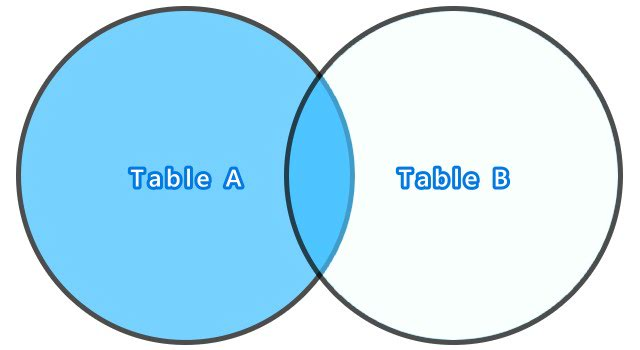``````使用指令與結果：
/*** JOIN TEST 03 ***/
SELECT *
FROM Table_A
LEFT OUTER JOIN Table_B
ON Table_A.cName = Table_B.cName
``````## 部份聯集：LEFT OUTER JOIN - Table_B IS NULL``````使用指令與結果：
/*** JOIN TEST 04 ***/
SELECT *
FROM Table_A
LEFT OUTER JOIN Table_B
ON Table_A.cName = Table_B.cName
WHERE Table_B.iIndex IS NULL
``````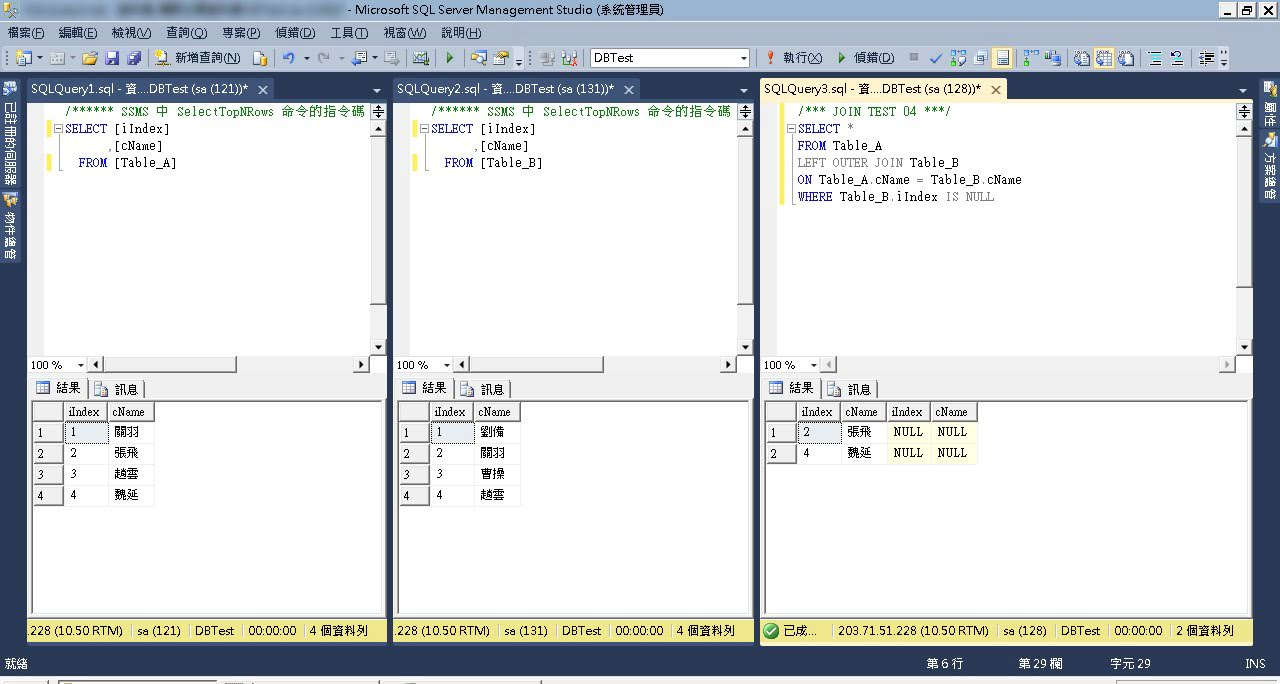## 部份聯集：FULL OUTER JOIN - Table_A OR Table_B IS NULL``````使用指令與結果：
/*** JOIN TEST 05 ***/
SELECT *
FROM Table_A
FULL OUTER JOIN Table_B
ON Table_A.cName = Table_B.cName
WHERE Table_A.iIndex IS NULL OR Table_B.iIndex IS NULL
``````